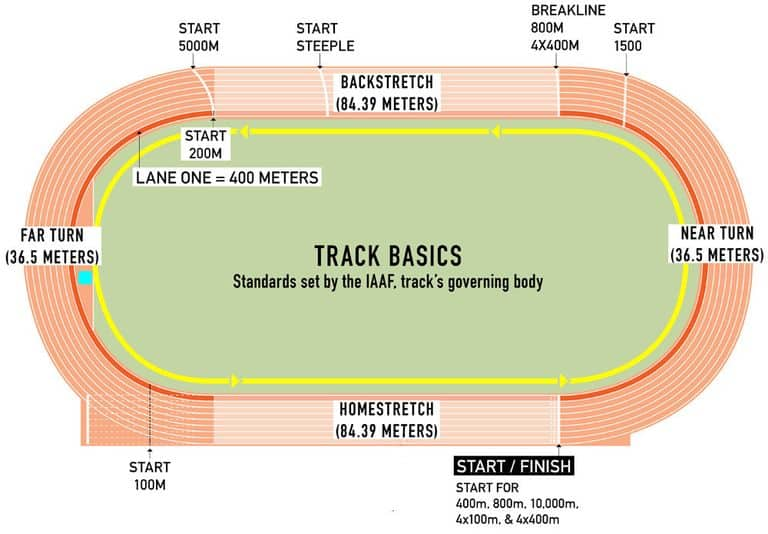# How Many Miles is 800 Meters?In the session, you will learn how to convert the meters to miles and miles to meters by standard rules of Mathematics. Initially, we will convert 800 meters to miles by using the conversion factor for your understanding.

## How Many Miles is 800 Meter?

800 meters exactly equal 0.497097 miles. However, it is roughly a half-mile. For example, 800m or half-mile is the shortest commonly run middle-distance running event.## Steps to Convert Meters to Miles

If you want to convert 800 meters to miles, you need to consider the following conversion factors.

1 meter = 0.00062137119 miles

You can use the above to convert any number of meters to miles by just the multiplication method. If you want to convert A meters to miles, you must know that you have A-meter copies of 1 meter. So you would multiply A by 0.00062137119 to get the answer in miles. Does it sound too straightforward? No worry about the enormous value 0.00062137119; we will also round off this for the sake of convenience in remembering.

In the current case A=800, let’s convert the distance of 800 meters to miles.

1 meter = 0.00062137119 miles

800 meters = 800 x 0.00062137119 miles

= 0.497096952 miles

## Is 800 Meter a Half Mile?

Yes, 800 meters is approximately equal to a half-mile. It is undoubtedly hard to remember the enormous value of 0.497096952 miles equivalent to 800 miles, but this is not disturbing; mathematics has a solution in such cases by rounding the values. Conventionally, 800 meters is associated with the half (0.5) mile. If we round 0.497096952 to the nearest tenth in one significant figure, we get 0.5 which is much easier to remember and deal with, saving time. We confirm that 800 meters are approximately equal to 0.5 or ½ miles.

## How Long is 800m Run?

800m long run is approximately equivalent to a half-mile. An excellent explanation for this is observed in the sport of track and field, and the most common and well-known runs are 400 meters, 800 meters, and 1600 meters. Most people use the term half a mile for the 800-meter run; however, we know that in actuality, this is slightly less than half a mile because technically 0.497 miles is not quite 0.5 miles.

## How Many Meters in a Half Mile?

We consider 800 meters in a half-mile, but exact mathematically calculated values slightly differ from the commonly known ones.

We use the conversion factor given as under:

1 mile = 1609.344 meters

Divide both sides by 2

½ mile = 1609.344/2

= 804.672 meters

Hence, we see 804.672 meters in a half-mile, not 800 meters.

## How Many Tenths of a Mile are 800 Meters?

To know the tenth of any number, we divide the number by 10 to get the answer. As we reached 0.497 or 0.50 miles per 800 meters, then tenths of a mile in 800 meters are 0.0497 or 0.05 miles.

## How Far are 800 Meters Down in the Ocean?

The nautical customary US unit for measuring ocean depth is fathom, now standardized to 6 feet or 72 inches. We can convert 800 meters to fathom, feet or inches by similarly using the conversion factors as we did for the meter to the mile.

We know that 800 meters down in the ocean is 0.497 miles (approximately 0.5 miles) deep, but what would be this depth in a fathom? Let’s convert 800 meters to other applied units using relevant conversion factors.

## How Many Fathoms in 800 Meters?

800 meters equals 437.6 fathoms where fathom represents a unit of length equal to six feet (1.8 meters), chiefly used in reference to the depth of water.

One meter = 0.547 fathom

800 meters = 800 x 0.547 fathoms

= 437.6 fathoms

We see that the 800-meter depth of oceans is 437.6 fathoms.

## How many feet in 800 meters?

In 800 meters there are 2625.6 feet. We can also note the depth of oceans in feet; as we discussed earlier, there are 6 feet in one fathom; we can choose 437.6 fathoms as a conversion factor to determine the feet in 800 meters by the following way.

800 meters = 437.6 fathoms

1 fathom = 6 feet

437.6 fathoms = 437.6 x 6

= 2625.6 feet

Inflation Is Eating IRA/401(k) Savings! How to Protect Your IRA/401(k) in Bad Times?